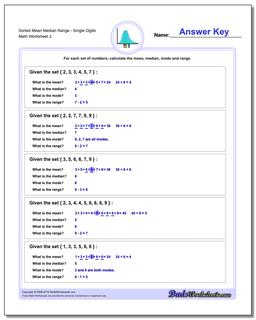# Math Worksheets: Mean, Median, Range: Mean, Median, Range: Sorted Mean Median Range - Single Digits (Second Worksheet)## Sorted Mean Median Range - Single Digits (Second Worksheet)

PropertyValue
DescriptionSorted Mean Median Range - Single Digits: Problem worksheets for finding the mean, median, mode and range given a set of numbers. These are basic problems suitable for "by-hand" calculations. The data in earlier problems are pre-sorted. (Second Worksheet)
Resource TypeWorksheet